Derivation of heat equation

## Linear Methods of Applied Mathematics Evans M. Harrell II and James V. Herod*

version of 8 September 1996

Newton articulated some principles of heat flow through solids, but it was Fourier who created the correct systematic theory. Inside a solid there is no convective transfer of heat energy and little radiative transfer, so temperature changes only by conduction, as the energy we now recognize as molecular kinetic energy flows from hotter regions to cooler regions. The first basic principle of heat is:

1. the heat energy contained in a material is proportional to the temperature, the density of the material, and a physical characteristic of the material called the specific heat capacity. In mathematical terms,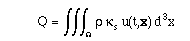(8.a1)

For the other principles of heat transfer, let us do some experiments with the following materials: a hot stove, some iron rods of different, relatively short lengths and various widths, and various ceramic rods of different lengths and widths. Since these will be thought experiments only, it will be safe to use a finger as the probe. Putting your finger right on the stove will convince you that the energy transfer is proportional to the difference in temperature between your finger and the stove. Using, if necessary, a different, undamaged finger, you will also find that the rate of heat transfer is inversely proportional to the length of an iron rod intervening between your finger to the stove (fixing the cross-sectional area). In other words, the rate of heat flow from one region to another is proportional to the temperature gradient between the two regions. You will probably also agree that the rate of heat flow will be proportional to the area of the contact; for example, a short pin with one end on a hot stove and the other touching your hand is preferable to putting the palm of your hand on a frying pan. Finally, a ceramic material on the stove being usually more pleasant to the touch than hot iron, we see that the rate of heat transfer depends on the material, as measured with a physical constant known as the heat conductivity. The second basic principle is thus:

2. The heat transfer through the boundary of a region is proportional to the heat conductivity,
to the gradient of the temperature across the region, and to the area of contact, so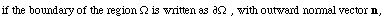then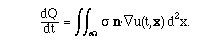If we differentiate eq. (8.a1) with respect to time and apply Gauss's divergence theorem to (8.a2), we find that dQ/dt can be expressed in two ways as an integral: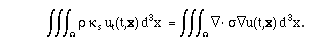Since the region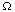can be an arbitrary piece of the material under study, the integrands must be equal at almost every point. If the material under study is a slab of a homogeneous substance, then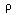,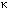s, andare independent of the position x, and we obtain the heat equation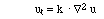,

where k =/s. Ordinary substances have values of k ranging from about 5 to 9000 cm2/gm (see table).

The one-dimensional heat equation

ut = k uxx

would apply, for instance, to the case of a long, thin metal rod wrapped with insulation, since the temperature of any cross-section will be constant, due to the rapid equilibration to be expected over short distances.